# Single Phase Matrix Converter Applications (Part 2)

A study of applications of Matrix Converter in single phase is presented. Single Phase Matrix Converter topology (SPMC) has many advantages. It is flexible and can replace many converter topologies by its wide applications as universal converter…Modulation techniques

Sinusoidal Pulse Width Modulation (SPWM)

Pulse Width Modulation (PWM) is a competent way of providing intermediary amount of electrical power between complete on and off. PWM is a comparatively recent technique, made practical by modern electronic power switches. The main benefit of carrier based SPWM is that the complication is very low and the dynamic response is also good for Matrix Converters.

Pulse width is a sinusoidal function of angular position of each cycle. This is done by comparing a triangular wave and a sinusoidal waveform of frequency as that is the input voltage generally. The pulses per cycle are decided by the ratio of the triangular carrier frequency to that of the modulating sinusoidal frequency. Modulation index is given by the ratio of amplitude of reference waveform to that of the carrier waveform. Modulation index= Ar / Ac, where Ar is the reference amplitude and Ac, the carrier amplitude. The SPWM conceptual diagram is shown in Figure 15.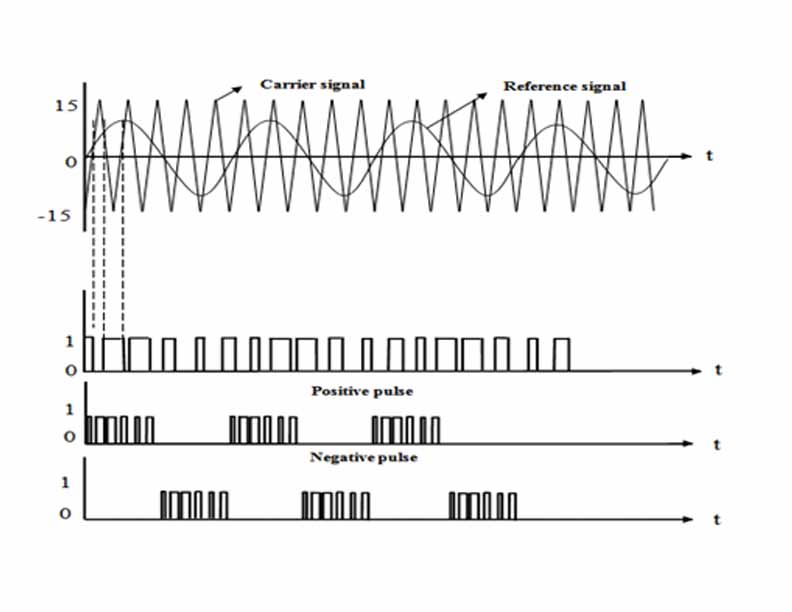Fig. 15. Carrier based Modulation Technique (SPWM)

The generation of Gate pulses via Sinusoidal Pulse Width Modulation could be easily understood from the Simulation diagram of MATLAB/Simulink, which is shown in Figure.16. From the above diagram it is observed that the PWM pulses are developed comparing the triangular waveform of the switching frequency with that of the sinusoidal waveform of the input supply frequency. Here the PWM is developed by unipolar voltage switching in which the pulses of unequal widths are generated. This train of pulses is again compared with the 50 Hz sinusoidal waveform to develop the pulses of switching frequency for first half cycle of the input to the converter. Similarly, the pulses train is again compared with the inverted 50 Hz sinusoidal waveform to develop the pulses for negative half cycle of the input.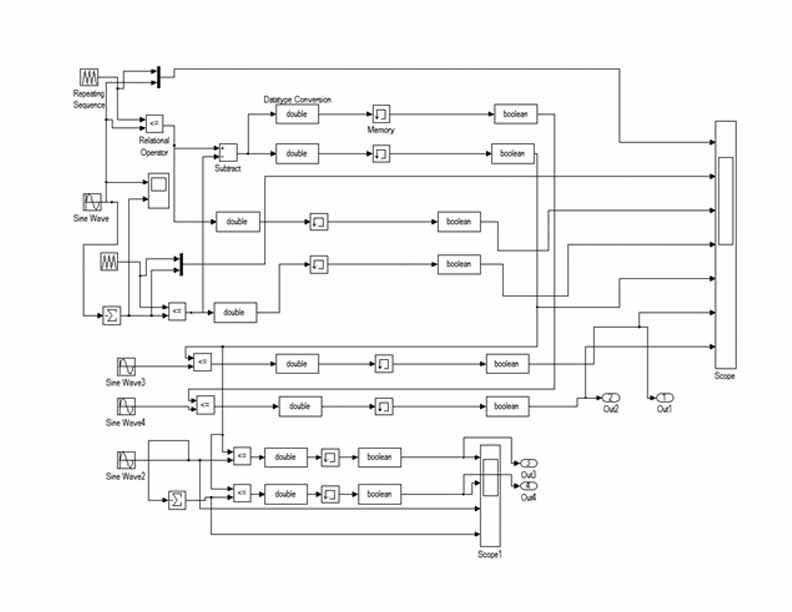Fig. 16. Simulation diagram of SPWM in MATLAB/Simulink

The Gate pulses for positive and negative cycles of the input for Matrix Converter as Cyclo-inverter operation is obtained by comparing the train of pulses with that of sinusoidal waveform of the required output frequency (say 100 Hz) for which the output of the Cyclo-inverter would be as desired (say 100 Hz in this case). This is shown in Figure 17 & 18 in which the PWM pulses are developed for the cycles of 50 Hz and 100 Hz respectively.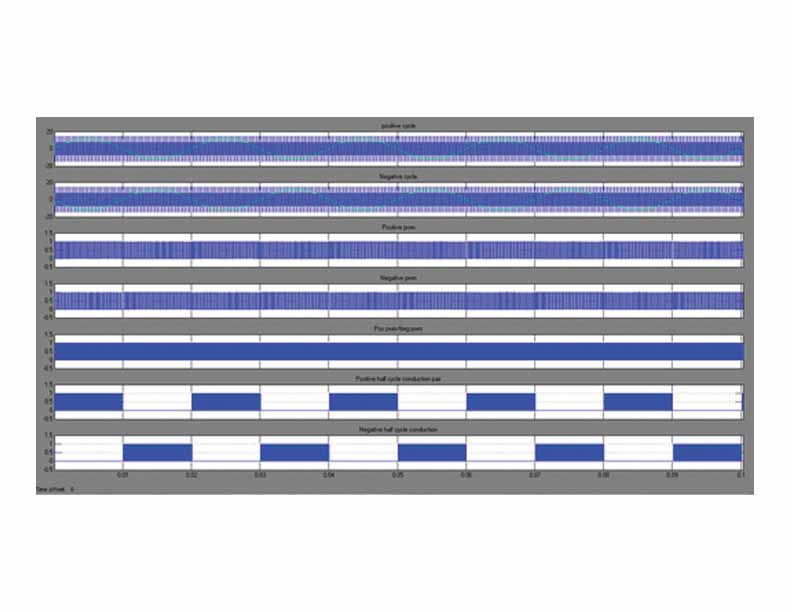Fig. 17. SPWM output (50Hz) in MATLAB/SimulinkFig. 18. SPWM generation (100Hz) for Cyclo-inverter operation of Matrix Converter

Selective Harmonic Elimination-Pulse Width Modulation (SHE-PWM)

SHE-PWM in one of the modulation methods in which a number of lower order harmonics can be eliminated or controlled effectively. It will thus help in the reduction of filter size, facilitates linear control of the output voltage and also the switching losses. The main objective of SHE technique is to compute the optimized switching angles for the fundamental value to reach its desired level and thus reducing the lower order harmonics. SHE method always results in multiple solutions for a set of nonlinear transcendental equations for the defined objective function. These equations are solved by Newton-Raphson method or theory of resultant polynomial technique. These solving techniques are quite complex and thus evolutionary algorithms such as Genetic Algorithm (GA), Ant Colony Optimization (ACO), Particle Swarm Optimization (PSO) have been employed.

Delta Modulation

In Delta modulation technique Sinusoidal reference wave is compared with a delta shaped carrier wave to synthesize the PWM. The carrier wave is allowed to oscillate within a defined band equally above and below the reference wave. An error signal is produced by comparing reference signal with that of carrier. This error signal is then quantized into either positive or negative level amplitude based on the polarity. The time duration between the successive levels is determined by the slope of the reference signal. The output binary pulses are produced by regular sampling of comparator output signal with the carrier frequency signal. The main advantage of delta modulation technique is that it requires simple circuitry with no feedback from external high power circuit, decreased low order harmonics.

Conclusion

It was shown that the single phase matrix converter can be realised as a universal applicable topology replacing many converters for their applications. The use of matrix converter in future reduces the need for learning varying converter topologies of power conversion for several applications. The SPMC, which is highly flexible in integration level can perform all the functions of generalised power electronics converters only by changing the input parameters and the gating signals. Single phase matrix converter can further be utilised for many potential applications like real and reactive power control of the system. Simple and efficient modulation techniques for single phase matrix converter are also reviewed.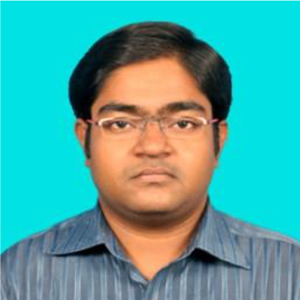K. Vijayakumar possesses an M.E. Degree from Anna University, Chennai. He is presently working as an Assistant Professor and pursuing research in the area of Power Electronics in the Department of EEE, Kalasalingam University, Krishnankoil – 626190, India.S. Kannan received PhD Degree from Madurai Kamaraj University. He is a Professor and Head of Electrical and Electronics Engineering, Ramco Institute of Technology, Rajapalayam – 626117, India.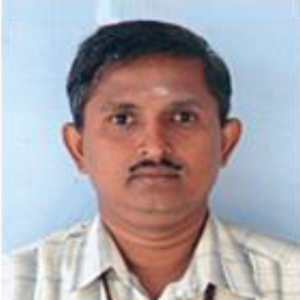S. Ponnayira Sundaravel is BE in Electrical and Electronics Engineering from Anna University, Chennai. He is presently working as Assistant Professor in the Department of EIE, Kalasalingam University, Tamil Nadu – 626190, India.